# do while Loop in C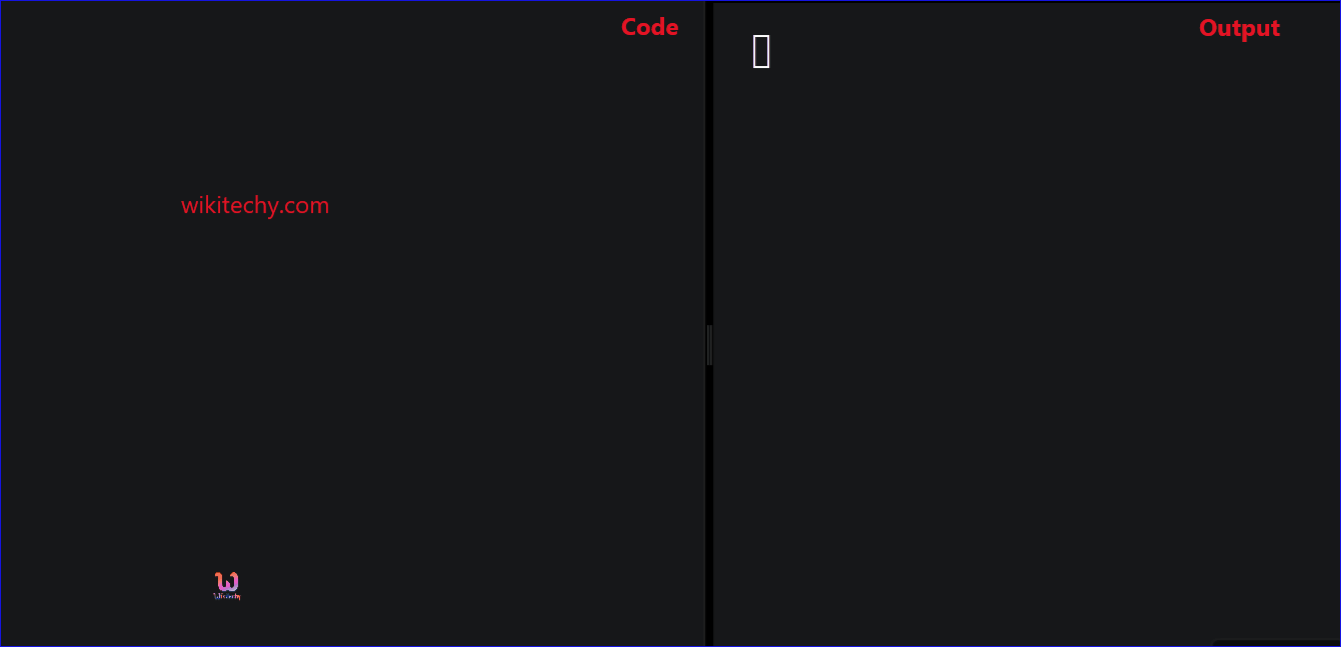Learn C - C tutorial - Do while loop in c - C examples - C programs

## do while Loop in C - Definition and Usage

• In C- Programming the do while statement is a looping statement.
• In C – Programming the do while Loop is executed at least one time then after executing the while loop for first time, then condition is checked.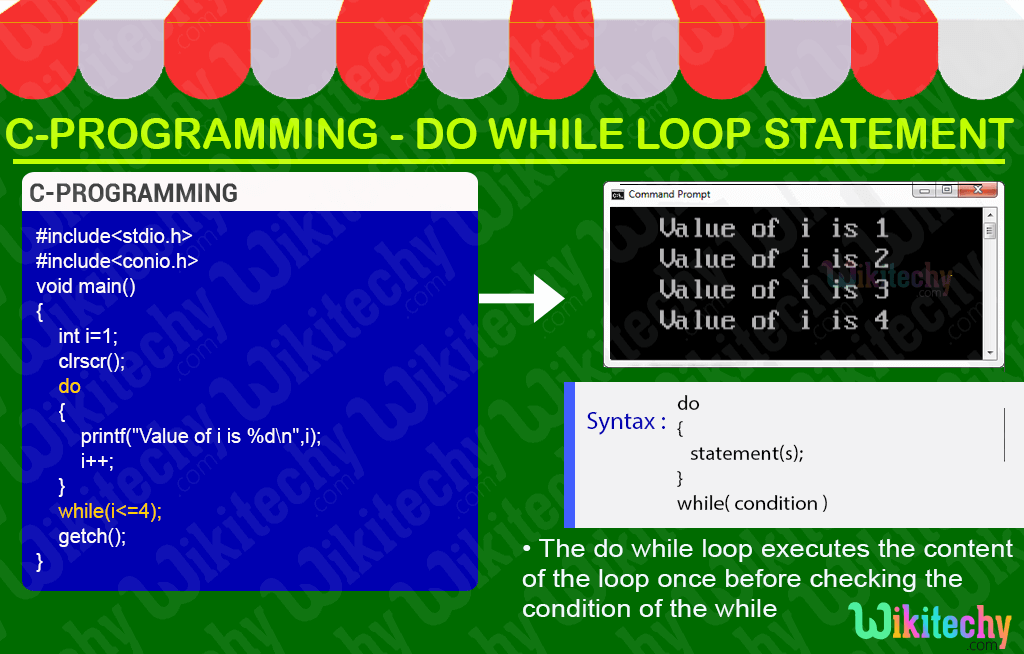## C Syntax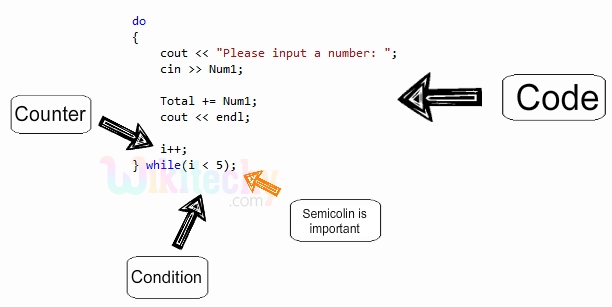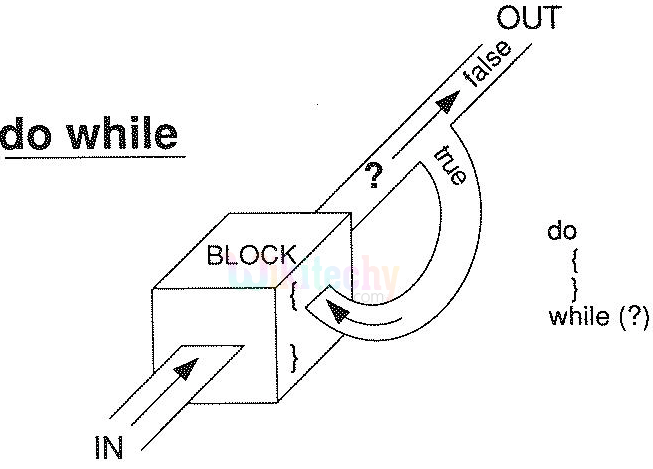## C Code - Explanation :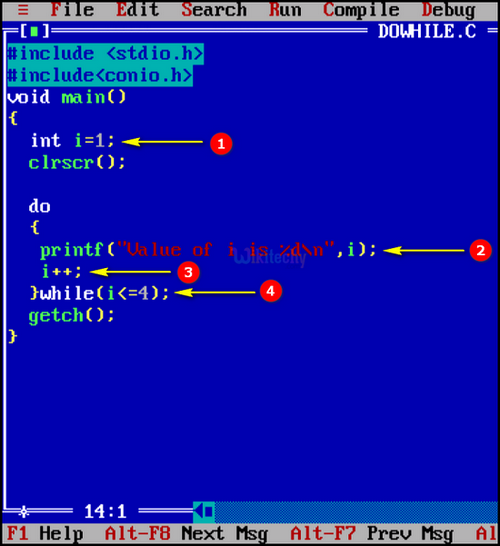1. Here in this statement we declare and initialize the value of “i=1”.
2. In this statement we print the value of “i” using printf statement.
3. In this statement we increment the value for “i”.
4. Here we check the condition value if the “i” is less than are equals to “4” for not.

## Sample Output - Programming Examples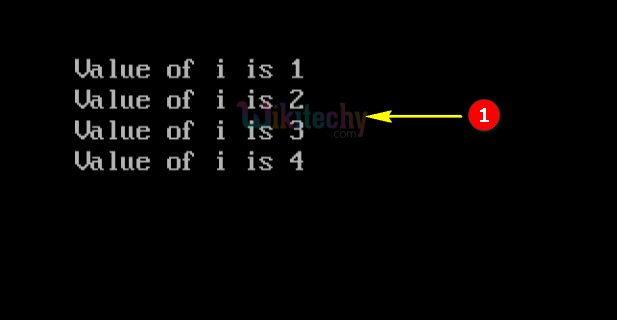1. Here in this output the “i” value is incremented by one for each do while loop execution which will be executed until the “i” value is less than are equal to “4”.